Testing Linear Hypotheses

Testing of linear hypotheses based on estimable functions is discussed in the section Test of Hypotheses in Chapter 3: Introduction to Statistical Modeling with SAS/STAT Software, and the construction of special sets of estimable functions corresponding to Type I–Type IV hypotheses is discussed in Chapter 15: The Four Types of Estimable Functions. In linear regression models, testing of general linear hypotheses follows along the same lines. Test statistics are usually formed based on sums of squares that are associated with the hypothesis in question. Furthermore, whenis of full rank—as is the case in many regression models—the consistency of the linear hypothesis is guaranteed.

Recall from Chapter 3: Introduction to Statistical Modeling with SAS/STAT Software, that the general form of a linear hypothesis for the parameters is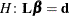, whereis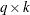,is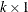, and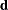is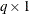. To test this hypothesis, you take the linear function with respect to the parameter estimates: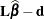. This linear function in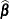has variance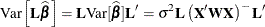The sum of squares due to the hypothesis is a simple quadratic form: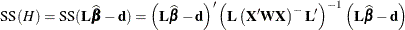If this hypothesis is testable, then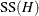can be used in the numerator of an F statistic: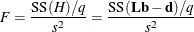Ifis normally distributed, which follows as a consequence of normally distributed model errors, then this statistic follows an F distribution with q numerator degrees of freedom and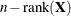denominator degrees of freedom. Note that it was assumed in this derivation thatis of full row rank q.